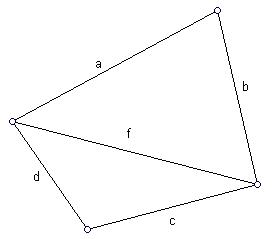favorite We need a little bit of your help to keep things running, click on this banner to learn more
Competitions

# HeronThe sides a, b, c, d and the diagonal f of a convex quadrilateral are given. Find the area of a quadrilateral, using the Heron’s formula that calculates the area of a triangle.

#### Input

In one line five real numbers are given: a, b, c, d, f (0 < a, b, c, d, f100). They are the lengths of segments as shown in the picture.

#### Output

Print one number - the area of a quadrilateral calculated with accuracy of 4 digits after the decimal point.

Time limit 1 seconds
Memory limit 128 MiB
Input example #1
```3 4 4 2 5
```
Output example #1
```9.7997
```
Source SFE-2010 Variant 26